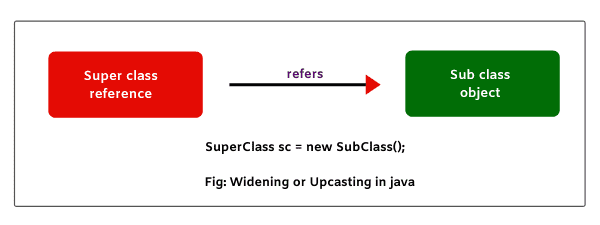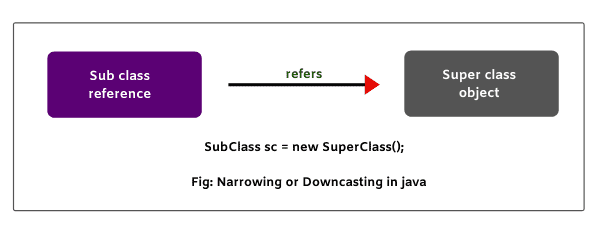# Upcasting and Downcasting in Java with Example

## What is Upcasting (Widening) in Java?

When the reference variable of super class refers to the object of subclass, it is known as widening or upcasting in java.

In other words, when the subclass object type is converted into superclass type, it is called widening or upcasting.

Look at the below figure, where superclass reference is pointing to the subclass object.Let’s understand it with the help of a suitable example. Suppose class One and class Two are related classes through inheritance. class One is a superclass and class Two is subclass of One.

1. Super class reference is used to refer to superclass object.

`One o = new One();`

In the above statement, class One’s reference o is pointing or referring to One’s object.

2. Similarly, sub class reference is used to point to sub class object.

`Two t = new Two();`

In this statement, class Two’s reference t is pointing to Two’s object.

In the above two statements, we do not need casting. This is because on the left side and at the right side of assignment operator (=), we have the same data type.

For example, in the first statement, o is a reference of class One. So, data type of o is One.

At right side of assignment operator, we have class One’s object. Data type of the class object is also One. Therefore, casting is not required in this case.

Till here, there is no need for casting. But when a reference of class refers to different class’s object, we need casting.

For example:

`One o = new Two(); // class One's reference o is pointing to class Two's object.`

In this statement, on the left side, the data type of reference o is One but on the right side, we got object whose data type is Two. So, in this case, we will need casting.

We will convert object’s data type into class O using cast operator. It can be done like this:

`One o = (One) new Two(); // Converting class Two's type into class One.`

Here, sub class object type has converted into super class type. This kind of conversion is called upcasting or widening in java.

If we do not use the cast operator in the above case, even we will not get any error message. Java compiler will do the implicit casting.

## Example Based on Upcasting (Widening)

Let’s take an example program to see the effect of upcasting where super class reference refers to sub class object.

Program code 1:

```package classCasting;
public class One
{
void m1()
{
System.out.println("m1 method in class One");
}
}
public class Two extends One
{
void m2()
{
System.out.println("m2 method in class Two");
}
}
public class Test
{
public static void main(String[] args)
{
One o = (One)new Two(); // Upcasting. Here, super class reference o refers to sub class object.
o.m1();
// o.m2(); // Compile-time error message.
}
}```
```Output:
m1 method in class One```

In this example program, superclass reference is pointing to subclass object. This means that sub class object type is converted into super class type. Now, observe that we are able to call m1() method of super class.

But when we are trying to call m2() method of sub class, we got error message: compile-time error. Hence, in upcasting or widening, we can call all methods of super class but not sub class methods.

Let’s take another program where we will override super class method in sub class and will observe that can we access super class method and sub class method.

Program code 2:

```package classCasting;
public class One
{
void m1()
{
System.out.println("m1 method in class One");
}
}
public class Two extends One
{
void m1()
{
System.out.println("m1 method in class Two");
}
}
public class Test
{
public static void main(String[] args)
{
One o = (One)new Two(); // Upcasting. Here, super class reference o refers to sub class object.
o.m1();
}
}```
```Output:
m1 method in class Two```

As you can observe in the program that it is possible to access sub class method but not super class method when super class method is overridden in sub class. This shows that you can access only 50% of the functionality.

## Downcasting (Narrowing) in Java

When subclass reference refers to super class object, it is called narrowing or downcasting in java. In other words, when sub class type is converted into super class type, it is called downcasting.

Look at the below figure where subclass reference is pointing to superclass object.For example:

`Two t = (Two) new One();`

Here, we are converting superclass object type into subclass type. Therefore, we need a compulsory cast operator. If we perform it directly, Java compiler will give compile-time error.

Let’s consider the above example program where we will do downcasting and see what happens?

Program code 3:

```package classCasting;
public class One
{
void m1()
{
System.out.println("m1 method in class One");
}
}
public class Two extends One
{
void m2()
{
System.out.println("m2 method in class Two");
}
}
public class Test
{
public static void main(String[] args)
{
Two t = (Two) new One(); // Downcasting. Here, subclass reference t refers to superclass object.
t.m1();
t.m2();
}
}```
```Output:
classCasting.One cannot be cast to classCasting.Two```

In the above example program, we have performed downcasting directly. Therefore, JVM is thrown an exception named ClassCastException at runtime.

So, in the case of downcasting, we cannot access any of the methods of super class or sub class. So, let’s understand how downcasting is possible in java?

## How Downcasting is possible?

To overcome this problem, we will have to modify the previous code. So, look at the following source code and modify it.

Program code 4:

```package classCasting;
public class One
{
void m1()
{
System.out.println("m1 method in class One");
}
}
public class Two extends One
{
void m2()
{
System.out.println("m2 method in class Two");
}
}
public class Test
{
public static void main(String[] args)
{
One o = new Two(); // Super class reference refers to sub class object.
Two t = (Two)o; // Converting super class reference type into sub class reference type.
t.m1(); // Calling m1 method using reference variable of sub class.
t.m2(); // Calling m1 method using reference variable of sub class.
}
}```
```Output:
m1 method in class One
m2 method in class Two```

In the above program, superclass reference type has converted into sub class reference type. Now, we can call both methods m1() and m2() using reference variable of sub class.

In the preceding code, you can observe that if we create an object of subclass, it is possible to access all the methods of super class and subclass. In this way, downcasting is possible in java.

Hope that this tutorial has covered almost all important points associated with upcasting and downcasting in java with example program.

I hope you will have understood to perform Java upcasting and downcasting nicely and enjoyed related programming.
Thanks for reading!!!Next ⇒ Method overriding in Java⇐ PrevNext ⇒